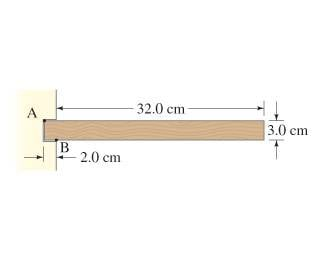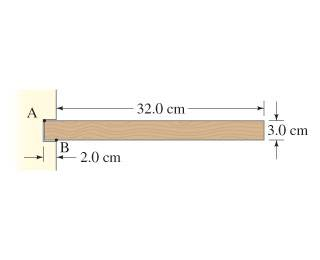# Torque problem

## Homework Statement

When a wood shelf of mass 7.7 kg is fastened inside a slot in a vertical support as shown in (Figure 1) , the support exerts a torque on the shelf. Assume that the slot exerts two vertical forces on the shelf, at points A and B.Determine the force exerted on the shelf by the support slot at A.

## The Attempt at a Solution

I know that the sum of the torques has to equal 0 and that the forces at A and CM is downwards, and upwards at B but I can't seem to get the correct answer (the correct answer is -566N)

What did you get for an answer?

Edit: I didn't get that answer either. But I don't have a lot of confidence in my answer because I know from experience that I make lots of mistakes. :)

Last edited:
CWatters
Homework Helper
Gold Member
I got the "right" answer. Use g=9.8 not 9.81 or 10.

Thanks @CWatters. Because of your post, I want back and reviewed my work and found my mistake. My mistake was greater than what would be caused by using different gravity values. And yes, 9.8 gives the right answer.

haruspex
Homework Helper
Gold Member
2020 Award

## Homework Statement

When a wood shelf of mass 7.7 kg is fastened inside a slot in a vertical support as shown in (Figure 1) , the support exerts a torque on the shelf. Assume that the slot exerts two vertical forces on the shelf, at points A and B.Determine the force exerted on the shelf by the support slot at A.

## The Attempt at a Solution

I know that the sum of the torques has to equal 0 and that the forces at A and CM is downwards, and upwards at B but I can't seem to get the correct answer (the correct answer is -566N)